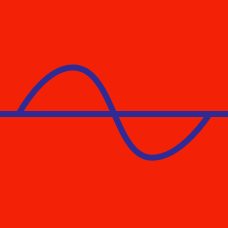Geometry

# Graphs of Trigonometric Functions - Problem Solving

Consider the intersection points of the two functions \begin{aligned} y&=\cos 26x \\ y&=k, \; (-1 in the domain $0 \leq x \leq \frac{3}{26}\pi$. If the $x$-coordinates of those points are $\alpha$, $\beta$ and $\gamma$ in an ascending order, the value of $\alpha+2\beta+\gamma$ can be expressed as $\frac{a}{b}\pi$, where $a$ and $b$ are coprime positive integers. What is the value of $a+b$?

If the minimum and maximum value of $y=\left| \sin x-\frac{1}{10}\right|+18$ are $a$ and $b$, respectively, what is the value of $a+10b$?

Consider the function $f(x)=a \cos bx+c,$ where $a>0$ and $b>0$. If the period of $f(x)$ is $\frac{2}{5}\pi$, the maximum value of $f(x)$ is $13$, and $f(\pi)=-11$, what is the value of $abc$?

Consider the function $f(x)=a|\cos bx|+c,$ where $a>0$ and $b>0$. If the period of $f(x)$ is $\frac{\pi}{7}$, the maximum value is $27$, and $f\left(\frac{\pi}{21}\right)=18$, what is the value of $a+b+c$?

Consider the function $f(x)=a \sin \left(\frac{x}{b}-\frac{\pi}{3}\right)-c,$ where $a>0$ and $b>0$. If the period of $f(x)$ is $34\pi$, the maximum value is $21$, and $f\left(\frac{17}{6}\pi\right)=0$, what is the value of $a+b-c$?

×